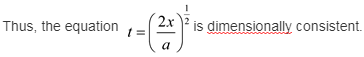# Which of the following equations are dimensionally consistent? (a) x = vt, (b) , (c) t = (2x/a)1/2

Which of the following equations are dimensionally consistent?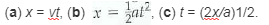(a)
The distance travelled by an object is
x = vt
Dimension of x is [L], dimension of v is [L]/[T] and dimension of t is [T].
Substitute [L] for x, [L]/[T] for v, and [T] for t,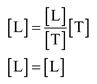The dimensions on both the sides are the same.
Thus, the equation x = vt is dimensionally consistent.

(b)
The distance travelled by an object isThe dimensions on both the sides are the same.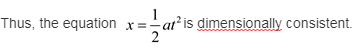( c)
Given equation is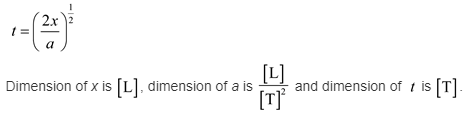Note that 2 is ignored in dimensional analysis because it has no dimensions.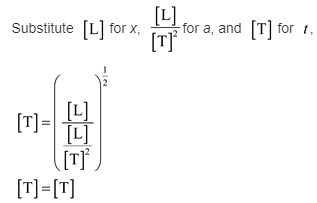The dimensions on both the sides are the same.